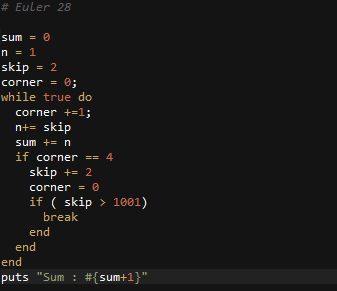# Ruby: Solution to Project Euler Problem # 28

http://projecteuler.net/problem=28

Number spiral diagonals

Starting with the number 1 and moving to the right in a clockwise direction a 5 by 5 spiral is formed as follows:

21 22 23 24 25
20  7  8  9 10
19  6  1  2 11
18  5  4  3 12
17 16 15 14 13

It can be verified that the sum of the numbers on the diagonals is 101.

What is the sum of the numbers on the diagonals in a 1001 by 1001 spiral formed in the same way?